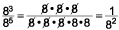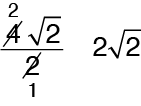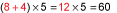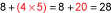# 1.2 Learn Basic Properties and Operations

 Site: KET Adult Education Course: Sample FF Lessons Book: 1.2 Learn Basic Properties and Operations
 Printed by: Guest user Date: Tuesday, May 17, 2022, 5:10 PM

## Description

This lesson will help you learn the basic properties and rules that govern math problem-solving.

## Lesson IntroductionTo play any sport, you have to first learn the rules. Beginners often think that rules exist to tell you what you can’t do, but rules actually define what you can do. Good athletes and coaches use the rules to develop strategies that will help their teams become winners.

As in sports, math has rules called properties. These properties define what we can do. Using them, we can create strategies that will help us become winners at problem solving and test taking.

In this lesson, you will learn the properties that allow us to manipulate expressions and solve equations. You will also see how these properties apply to operations.

The math skills that you learn in this lesson will help you make the right decisions when you are solving an algebra problem. Take time to learn the properties so that you can see how math works.

## Properties

The commutative property applies to addition and multiplication. It states that you can add or multiply numbers in any order without changing the result.

Note: We use letters when we write a property so that it is clear that the property will work for all numbers.

 Commutative property $$a + b = b + a$$ and $$ab = ba$$

### Examples

1.

We can add the numbers in any order, and the result will be 5.

2.

Changing the order of the numbers being multiplied doesn’t change the answer.
Remember, subtraction and division are not commutative operations. Changing the order changes the result.

The associative property applies when we are adding or multiplying more than two numbers. It states that we can group the numbers however we would like without changing the result.

In the property, parentheses show which operation is performed first.

 Associative property $$(a + b) + c = a + (b + c)$$ and $$(ab)c = a(bc)$$

### Examples

 $$\begin{array}{l}\;\;\;( - 7 + 4) + 6\\ = - 3 + 6\\ = 3 \end{array}$$ and $$\begin{array}{l}\;\;\;\; - 7 + (4 + 6)\\ = - 7 + 10\\= 3\end{array}$$ The result is 3 no matter how the numbers are grouped.In this example, the numbers in red were multiplied first.

It is often helpful to group the numbers with like signs. When adding or multiplying many numbers, make a group of positive numbers and a group of negative numbers. After finding the sum or product of the groups separately, combine the results.

The distributive property can be used when a number is multiplied by a sum or a difference. Instead of adding or subtracting first, we can multiply each number inside the parentheses by the number outside the parentheses. Then add or subtract.

 Distributive property $$a(b + c) = ab + ac$$ $$a(b - c) = ab - ac$$

### Example

What does $$2(7 + 9)$$ equal?

 One way to solve the problem is to add first and then multiply by 2. $$2(7 + 9) = 2(16) = 32$$ Another way is to use the distributive property. Multiply each term inside the parentheses by 2 and then add. $$\begin{array}{c}2(7 + 9) = 2(7) + 2(9)\\\,\,\,\,\,\,\,\,\,\,\, \,\,=14 + 18\\ = 32\end{array}$$

### Example

What does $$5(12 - 3)$$ equal?

 Without the distributive property: $$\,5(12 - 3) = 5(9) = 45$$ With the distributive property: $$\begin{array}{c}5(12 - 3) = 5(12) - 5(3)\\ \,\,\,\;\;\;\;\;\; = 60 - 15\\ = 45\end{array}$$

In a multiplication problem, the numbers being multiplied are called factors. In the expression 4(9 + 2), the factors are 4 and (9 + 2). We can work backward using the distributive property to find the factors in an expression.

### Example

Which of the following expressions is equal to $$5(15) - 5(8)$$?

#### Test Tip

Equal expressions have the same value when all of the operations are completed.

A. $$(15 - 5) - (8 - 5)$$
B. $$(15 - 5) + (8 - 5)$$
C. $$5(15 - 8)$$
D. $$5(15 + 8)$$

The correct answer is C. In the original expression, there are two terms: 5(15) and 5(8). Both terms have 5 as a factor, so we can factor out the 5, removing it from the terms. Then we write the numbers that are left as a subtraction inside parentheses.

.Guest users do not have permission to interact with embedded questions.

## Exponents

In algebraic expressions and equations, we often see numbers or variables raised to a power. The power is represented by an exponent, a small number written above and to the right of a base.

In the example below, 6 is the base and 3 is the exponent. The base tells the factor to be multiplied. The exponent tells how many times the base appears in the multiplication problem.

### Example

$${6^3} = 6 \times 6 \times 6 = 216$$

Since the powers 2 and 3 are often used, we have special words for them. A number raised to the second power is said to be “squared.”

### Example

“5 squared” means $${5^2}$$, which equals $$5 \times 5 = 25$$

A number raised to the third power is said to be “cubed.”

### Example

“4 cubed” means $${4^3}$$, which equals $$4 \times 4 \times 4 = 64$$

Some students make the mistake of thinking that squaring a number is the same as doubling it. It isn’t. Doubling a number multiplies the number by 2. (10 doubled equals 20.) Squaring a number multiplies that number by itself: $${10^2} = 100$$.

.

Numbers and variables with exponents are easy to work with if we follow the laws of exponents.

### The Laws of Exponents

1. To multiply numbers with the same base, add the exponents: $${a^m} \times {a^n} = {a^{m + n}}$$

### Examples

$${9^2}\cdot\,\,{9^3} = {9^{2 + 3}} = {9^5}$$$${3^4}\cdot \,\,{3^7} = {3^{4 + 7}} = {3^{11}}$$

2.
To divide numbers with the same base, subtract the exponents:  $${a^m} \div \;\,{a^n} = \;\,{a^{m - n}}$$

### Examples

$${4^5} \div {4^3} = {4^{5 - 3}} = {4^2}$$

3. To raise a number with an exponent to a power, multiply the exponents: $${({a^m})^n} = {a^{m \cdot n}}$$

### Examples

$${\left( {{7^2}} \right)^4} = {7^{2\,\cdot \,4}} = {7^8}$$$${\left( {{{10}^3}} \right)^7} = {10^{3\,\cdot \,7}} = {10^{21}}$$

The powers of ten form patterns that can help you understand exponents. In the examples, pay close attention to the pattern formed by the zeros.

### Examples

 $$\begin{array}{c}\,\,\,\,\,\,\,\,\,\,\,\,{10^4} = 10,000\\\,\,\,\,\,\,\,\,\,{10^3} = 1,000\\\,\,\,\,\,{10^2} = 100\\\,\,\,{10^1} = 10\\{10^0} = 1\end{array}$$

Did you notice? The powers of ten are very easy to figure out. Each is equal to 1 followed by the number of zeros shown by the exponent.

This pattern also reveals what will happen when we raise a number to the first or zero power.

Any number raised to a power of 1 doesn’t change: $${a^1} = a$$
Any number raised to a power of 0 equals 1: $${a^0} = 1$$

.

Exponents can also be negative numbers. You already know that to divide exponents, you should subtract.

### Examples

 $${\textstyle{{{8^3}} \over {{8^5}}}} = {8^{3 - 5}} = {8^{ - 2}}$$ You can also think of it this way:You can see that $${8^{ - 2}} = {\textstyle{1 \over {{8^2}}}}$$ . When you see a negative exponent, move the base and its exponent to the other side of the fraction bar and change the sign of the exponent.

### Examples

 $${3^{ - 2}} = {\textstyle{1 \over {{3^2}}}}$$ $${\textstyle{1 \over {{4^5}}}} = {4^{ - 5}}$$ $${\textstyle{{{2^{ - 2}}} \over {{9^{ - 3}}}}} = {\textstyle{{{9^3}} \over {{2^2}}}}$$
.Guest users do not have permission to interact with embedded questions.Guest users do not have permission to interact with embedded questions.

A radical , also called a root, is the opposite of an exponent. Finding a is the opposite of squaring a number. The bracket symbol $$\sqrt {\;}$$ indicates a square root.

### Examples

 $${8^2} = 64$$ 8 squared is 64. $$\sqrt {64} = 8$$ The square root of 64 is 8.

You may have noticed that –8 could also be the square root of 64 because $$( - 8)( - 8) = 64$$. Most real-life situations require the positive root, but in special situations both roots may be needed. We can show both roots in this way: $$\sqrt {16} = \pm 4$$, which means “the square root of 16 is plus or minus 4.”

A cube root is the opposite of raising a number to the third power. Anis written in the angle of the bracket to show which root is meant.

### Examples

 $$\sqrt{8} = 2$$ The cube root of 8 is 2 because $${2^3} = 8$$. $$\sqrt{{625}} = 5$$ The fourth root of 625 is 5 because $${5^4} = 625$$.

A cube root can be negative: $$\sqrt { - 27} = - 3$$ because $$( - 3)( - 3)( - 3) = - 27$$.

Without a calculator, the exact square root of a number may difficult to find. However, you can find the approximate square root of a number by thinking of the square numbers closest to your number. For example, you know that the square root of 12 must be between 3 and 4 because 12 falls between $${3^2} = 9$$ and $${4^2} = 16$$.

### Example

Which of the following is the best estimate for the square root of 70?

A. between 7 and 8
B. between 8 and 9
C. between 9 and 10
D. between 30 and 40

The number 70 is greater than $${8^2} = 64$$ and less than $${9^2} = 81$$, so the correct choice is B. Using a calculator, we see that the square root of 70 is about 8.37.

A represents the range of numbers from $${7^2} = 49$$ to $${8^2} = 64$$.

C represents the range of numbers from $${9^2} = 81$$ to $${10^2} = 100$$.

D represents the range of numbers from 900 to 1,600. If you chose D, you may have divided 70 by 2. You need to think, “What number multiplied by itself is 70?”

In algebra, answers should be written in simplest form. We can simplify a square root by looking for perfect square factors of the number inside the bracket.

### Example

Simplify $$\sqrt {75}$$.

 $$\sqrt {75} = \sqrt {25} \,\cdot \,\sqrt 3$$ The first step is to write factors for 75 using at least one perfect square. 25 is a perfect square because $${5^2} = 25$$. $$\sqrt {25} \,\cdot \,\sqrt 3 = 5\sqrt 3$$ Find the square root of 25 and write this number in front of the remaining factor $$\sqrt 3$$.

AnswerIn simplest form, $$\sqrt {75} = 5\sqrt 3$$.

Sometimes you can factor a number with more than one perfect square factor.

### Example

Simplify $$\sqrt {72}$$.

 $$\sqrt {72} = \sqrt 9 \,\cdot \,\sqrt 4 \,\cdot \,\sqrt 2$$ If you didn’t notice that 36 is a factor of 72, you can factor 72 using perfect squares 9 and 4. $$\begin{array}{c}\sqrt 9 \,\cdot \,\sqrt 4 \,\cdot \,\sqrt 2 \\ = \,\,3\,\cdot \,2\,\cdot \,\sqrt 2 \\ = 6\sqrt 2 \,\,\,\,\,\,\,\,\,\,\,\,\,\,\,\,\end{array}$$ Multiply the square roots of 9 and 4. Write the product 6 in front of $$\sqrt 2$$ to show that $$\sqrt 2$$ is multiplied by 6.

AnswerIn simplest form, $$\sqrt {72} = 6\sqrt 2$$.

In algebra, a fraction could contain a radical. Whether the radical is in the numerator or the denominator, make sure it is simplified.

When a radical is in the denominator, we usually use multiplication to remove the radical. We will use the idea that for any number, the square of a square root equals the number itself: $$\sqrt a \,\cdot \,\sqrt a \, = a$$.

### Example

Simplify $${\textstyle{4 \over {\sqrt 2 }}}$$.

 $${\textstyle{4 \over {\sqrt 2 }}}\,\,\cdot \,{\textstyle{{\sqrt 2 } \over {\sqrt 2 }}} = {\textstyle{{4\sqrt 2 } \over 2}}$$ We want to remove the radical from the denominator. We can multiply the fraction by $${\textstyle{{\sqrt 2 } \over {\sqrt 2 }}}$$ without changing the value of the fraction.We can divide both the top and bottom by 2. In simplest form, $${\textstyle{4 \over {\sqrt 2 }}} = 2\sqrt 2$$.Guest users do not have permission to interact with embedded questions.Guest users do not have permission to interact with embedded questions.

## Order of Operations

When an expression has more than one operation, the order that you perform the operations could affect the answer.

 Consider this expression: $$8 + 4 \times 5$$ If you add first, the answer is 60.If you multiply first, the answer is 28.Mathematicians have agreed upon a set of rules for solving problems with more than one operation. The rules are called the order of operations.

 The Order of Operations Perform operations in parentheses or other grouping symbols. Evaluate exponents or roots. Working from left to right, perform multiplication and division steps. Working from left to right, perform addition and subtraction steps.

To remember these steps, many people memorize this acronym, or memory trick: Please Excuse My Dear Aunt Sally (PEMDAS). The letters stand for parentheses, exponents, multiplication, division, addition, and subtraction.

Watch the video about applying the order of operations.

In the example below, notice how the fraction bar is used as a grouping symbol. A fraction is used to show division, but if there is an operation in the top or bottom of the fraction, you need to perform that operation first before you divide.

Other grouping symbols include brackets, the radical symbol, and the bars used to indicate absolute value.

### Example

Find the value of the expression: $${\textstyle{{4 + 5} \over { - 3}}}\,\,\cdot \,\,( - 5 + 3)$$.

 Step 1 Do the two operations in grouping symbols.Step 2 Then multiply. There are no other operations. Then multiply.

Answer$${\textstyle{{4 + 5} \over { - 3}}}\,\,\cdot\,\,( - 5 + 3) = 6$$

In this example, a radical is used as a grouping symbol. Remember that a radical, or root, is part of the second step in the order of operations.

### Example

Find the value of the expression: $$\sqrt {6 + 10} \,\, + \,2\,\,\cdot \,\,{3^2}$$

 Step 1 Do the operation in the radical first. $$\begin{array}{l}\;\;\;\,\sqrt {6 + 10} \,\, + \,2\,\,\cdot \,\,{3^2}\\ = \sqrt {16} + 2\,\,\cdot \,\,{3^2}\\ = 4 + 2\,\,\cdot \,\,9\\ = 4 + 18\\ = 22\end{array}$$ Step 2 Evaluate the root $$\sqrt {16}$$ and the exponent $${3^2}$$. Step 3 Multiply: $$2\,\,\cdot \,\,9$$ Step 4 Add: $$4 + 18$$

Answer$$\sqrt {6 + 10} \,\, + \,2\,\,\cdot\,\,{3^2} = 22$$

You won’t always need every step in the order of operations, but it’s a good idea to think through the steps each time you evaluate an expression to make sure your result is the correct one.Guest users do not have permission to interact with embedded questions.Guest users do not have permission to interact with embedded questions.